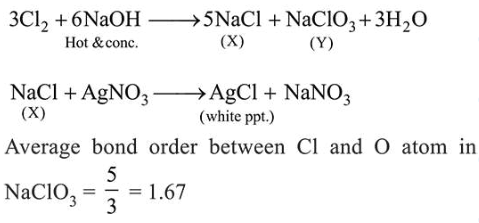# Chlorine reacts with hot and concentratedQuestion:

Chlorine reacts with hot and concentrated $\mathrm{NaOH}$ and produces compounds $(X)$ and $(Y)$. Compound $(X)$ gives white precipitate with silver nitrate solution. The average bond order between $\mathrm{Cl}$ and $\mathrm{O}$ atoms in $(Y)$ is __________________

Solution:

(1.67)